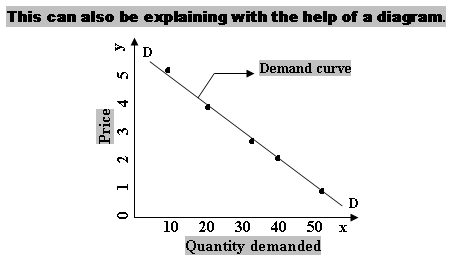SSC BOARD PAPERS IMPORTANT TOPICS COVERED FOR BOARD EXAM 2024

### State and explain the law of demand with its assumptions.

THE LAW OF Demand.

Meaning: -The Law of demand establishes the functional relationship between the Price of a commodity and the quantity of that commodity demanded at different prices, assuming other factors remaining constant.

When the price of a commodity rises, demand for it falls and when the price of the commodity falls, demand rises. So less quantity is demanded at higher prices and more quantity is demanded at lower prices. There exist inverse relationship between price and quantity demanded of a commodity.

Definition: -According to Marshall the law of demand, is defined asOther things being equal, the quantity of a commodity demanded varies inversely with its price.”

Symbolically, the Law of demand can be expressed as follows:

Dx = f (Px)
Where, D = stands for the demand for commodity X
X stands for the commodity demanded
F stands for function of
Px stands for the price of the commodity X

We can explain this law with the help of a schedule and a diagram.

 Price (Rs.) Quantity Demanded 1 2 3 4 5 50 40 30 20 10

The Schedule shows that with an increase in Price the quantity demanded is decreasing. It indicates inverse relationship between the two variables price and quantity demanded. When the price is Re. 1 the consumer demand 50 units and when the price rises to Rs. 5 he demands the least that is 10 units.In the above diagram X-axis represents quantity demanded and Y-axis represents price. Various points from the schedule are plotted on the graph, joint those points we will be getting demand curve. DD is the demand curve which slopes downward from left to right indicating inverse relationship between price and Quantity demanded. This happens when the price is more, demand is less and when price is less, and demand is more.

### Assumptions to the law of DEMAND

1.      No change in income level of the consumer:if the law of demand is to operate, consumers’ income remain constant. If there is rise in income, people may demand more at higher prices.

2.      No change in Consumer Taste, Preference and habit: -It is assumed that consumers’ habits, preferences, attitudes etc. remain unchanged. In other words, there is no change in consumers’ choice for the product.

3.      No change in prices of substitute goods: -A change in the price of substitutes will affect the demand for the commodity. If the price of substitutes fall much more the price of the commodity, people may not demand more of that commodity even at lower prices.

4.      No introduction of any substitutes: -it is also assumed that there is no introduction of any new substitutes in the market.

5.      No anticipation of price change in future: -It is also assumed that people do not anticipate any further change in the price in the near future. If people expect a further rise in price, they may demand more even the existing high price. Similarly, if people expect a further fall in price, they may not buy more even at the existing low price.

6.      No change in Size, sex and age composition of the population: -It is assumed that the size and composition of the population remain unchanged. After all, it is the population of a country that constitute the total market demand for a product. So any change in the size and composition of population of a country affect the total market demand for a product.

7.      No change in the taxation policy of the government: - Government policies on direct and indirect taxes have great impact on demand for various goods and services. Therefore, it is assumed that there is no change in government policy on taxation.